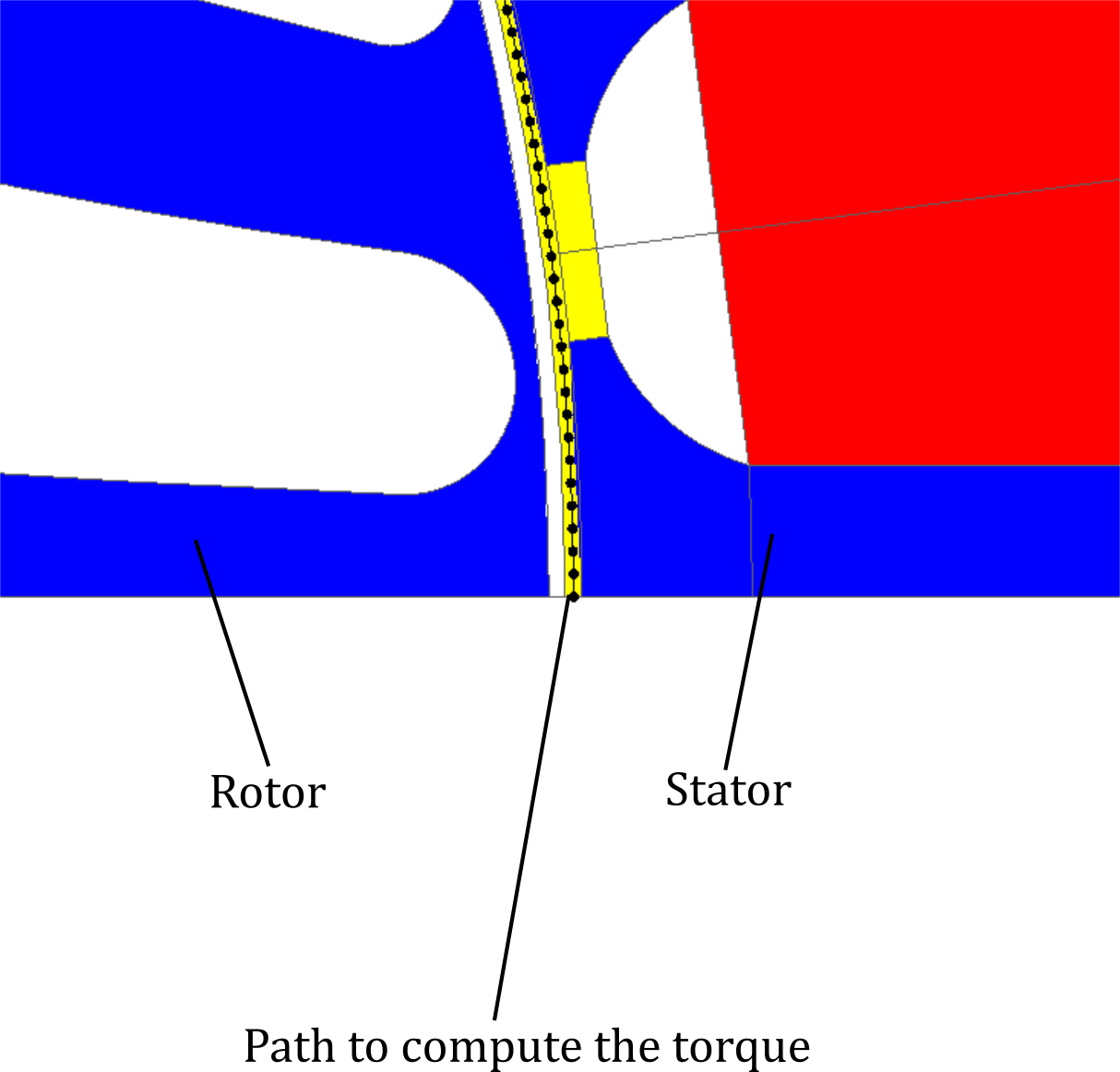# Responses

## Introduction

In the data tree of Flux the node Solver > Optimization > Responses allows the user to define physical quantity that will be optimized during the optimization process engaged by Flux. The short list of the responses is given below:
Table 1. Table summarizing all the responses available in Flux
Physical quantity to optimize Formula Entity
Flux flowing through faces
• L : Depth of the domain
• S : Area of the coil
• Az : Magnetic vector potential in Z direction
On a face belonging to a coil conductor region
Flux flowing through a line
• L : Depth of the domain
• Az : Magnetic vector potential in Z direction
• n1 and n2 the end nodes of the line where the flux is computed
On a line
Sum of the fluxes of selected coils
• n : Number of selected coils
• L : Depth of the domain
• Nsi : Winding function of the associated coil
• Az : Magnetic vector potential in Z direction
On one or several coil conductor components
Force computed with the integral of Pn on a path
• L : Depth of the domain
• Bn : Normal magnetic flux density
• Bt : Tangential magnetic flux density
• µ0 : Air magnetic permeability
Based on the Maxwell tensors approach, this method requires a path in a front of a piece of iron (plunger for an actuator, stator tooth ...)
Attention: This method is valuable only along a path in a air or vaccum region.
Torque computed with the integral of Pt on a path
• L : Depth of the domain
• p : Number of periodicities
• R : Radius of the path
• Bn : Normal magnetic flux density
• Bt : Tangential magnetic flux density
• µ0 : Air magnetic permeability
Based on the Maxwell tensors approach, this method requires a path in the airgap of a rotating machine, on the stator side as depicted in th following picture:Attention: This method is valuable only along a path in a air or vaccum region.
Volume of 2D faces   On faces